Students can download Maths Chapter 1 Relations and Functions Unit Exercise 1 Questions and Answers, Notes, Samacheer Kalvi 10th Maths Guide Pdf helps you to revise the complete Tamilnadu State Board New Syllabus, helps students complete homework assignments and to score high marks in board exams.

## Tamilnadu Samacheer Kalvi 10th Maths Solutions Chapter 1 Relations and Functions Unit Exercise 1

Question 1.
If the ordered pairs (x2 – 3x, y2 + 4y) and (-2, 5) are equal, then find x and y.
(x2 – 3x, y2 + 4y) = (-2, 5)
x2 – 3x = -2
x2 – 3x + 2 = 0
(x – 2) (x – 1) = 0
x – 2 = 0 or x – 1 = 0
x = 2 or 1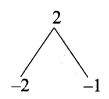y2 + 4y = 5
y2 + 4y – 5 = 0
(y + 5) (y – 1) = 0
y + 5 = 0 or y – 1 = 0
y = -5 or y = 1
The value of x = 2, 1
and 7 = -5, 1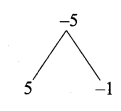Question 2.
The Cartesian product A × A has 9 elements among which (-1, 0) and (0, 1) are found. Find the set A and the remaining elements of A × A.
Solution:
A = {-1, 0, 1}, B = {1, 0, -1}
A × B = {(-1, 1), (-1, 0), (-1, -1), (0, 1), (0, 0), (0, -1), (1, 1), (1, 0), (1, -1)}Question 3.
Given that f(x) = $$\left\{\begin{array}{rl} {\sqrt{x-1}} & {x \geq 1} \\ {4} & {x<1} \end{array}\right.$$.
Find
(i) f(0) (ii)f (3) (iii) f(a + 1) in terms of a.(Given that a > 0)
f(x) = $$\sqrt { x-1 }$$ ; f(x) = 4
(i) f(0) = 4
(ii) f(3) = $$\sqrt { 3-1 }$$ = $$\sqrt { 2 }$$
(iii) f(a + 1) = $$\sqrt { a+1-1 }$$ = $$\sqrt { a }$$

Question 4.
Let A = {9, 10, 11, 12, 13, 14, 15, 16, 17} and let f: A → N be defined by f(n) = the highest prime factor of n ∈ A. Write f as a set of ordered pairs and find the range of f.
Solution:
A = {9, 10, 11, 12, 13, 14, 15, 16, 17}
f: A → N
f(n) = the highest prime factor of n ∈ A
f = {(9, 3), (10, 5), (11, 11), (12, 3), (13, 13), (14, 7), (15, 5), (16, 2), (17, 17)}
Range = {3, 5, 11, 13, 7, 2, 17}
= {2, 3, 5, 7, 11, 13, 17}Question 5.
Find the domain of the function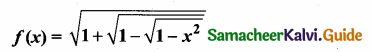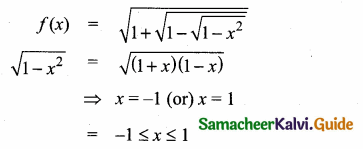Domain of f(x) = {-1, 0, 1}

Question 6.
If f(x)= x2, g(x) = 3x and h(x) = x – 2 Prove that (fog)oh = fo(goh).
Solution:
f(x) = x2
g(x) = 3x
h(x) = x – 2
(fog)oh = x – 2
LHS = fo(goh)
fog = f(g(x)) = f(3x) = (3x)2 = 9x2
(fog)oh = (fog) h(x) = (fog) (x – 2)
= 9(x – 2)2 = 9(x2 – 4x + 4)
= 9x2 – 36x + 36 ……………. (1)
RHS = fo(goh)
(goh) = g(h(x)) = g(x – 2)
= 3(x – 2) = 3x – 6
fo(goh) = f(3x – 6) = (3x – 6)2
= 9x2 – 36x + 36 ………….. (2)
(1) = (2)
LHS = RHS
(fog)oh = fo(goh) is proved.Question 7.
Let A= {1,2} and B = {1,2,3,4}, C = {5,6} and D = {5,6,7,8}. Verify whether A × C is a subset of B × D?
Given A = {1, 2}
B = {1, 2, 3, 4}
C = {5,6}
D = {5,6, 7,8}
A × C = {1,2} × {5,6}
= {(1,5) (1,6) (2, 5) (2, 6)}
B × D = {1,2, 3, 4} × {5, 6, 7, 8}
= {(1,5) (1,6) (1,7) (1,8)
(2, 5) (2, 6) (2,7) (2, 8)
(3, 5) (3, 6) (3, 7) (3, 8)
(4, 5) (4, 6) (4, 7) (4, 8)}
∴ A × C ⊂ B × D
Hence it is verified

Question 8.
If f(x) = $$\frac{x-1}{x+1}, x \neq 1$$ Show that
f(f(x)) = – $$\frac { 1 }{ x }$$, Provided x ≠ 0.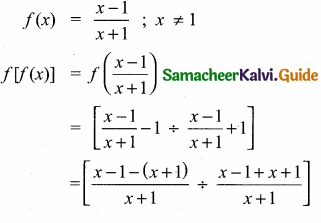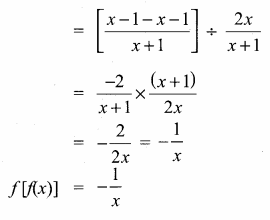Question 9.
The functions f and g are defined by f{x) = 6x + 8; g(x) = $$\frac { x-2 }{ 3 }$$
(i) Calculate the value of gg $\frac { 1 }{ 2 }$
(a) Write an expression for gf (x) in its simplest form.
f(x) = 6x + 8 ; g(x) = $$\frac { x-2 }{ 3 }$$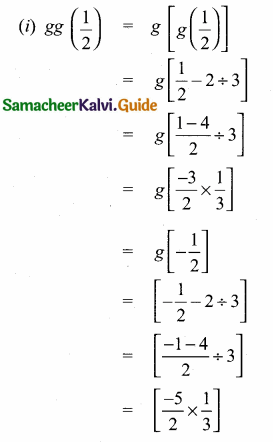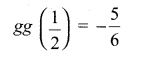Question 10.
Write the domain of the following real functions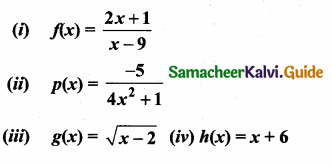(i) f (x) = $$\frac { 2x+1 }{ x-9 }$$
If the denominator vanishes when x = 9
So f(x) is not defined at x = 9
∴ Domain is x ∈ [R – {9}]

(ii) if p(x) = $$=\frac{-5}{4 x^{2}+1}$$
p(x) is defined for all values of x. So domain is x ∈ R.

(iii) g(x) = $$\sqrt { x-2 }$$
When x < 2 g(x) becomes complex. But given “g” is real valued function.
So x > 2
Domain x ∈ (2, α)

(iv) h (x) = x + 6
For all values of x, h(x) is defined. Hence domain is x ∈ R.